# Selina Concise Solutions for Chapter 11 Algebraic Expressions (including Operations on Algebraic Expressions) Class 8 ICSE Mathematics

##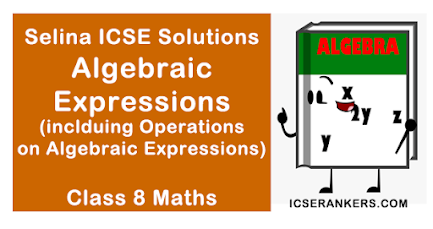### Exercise 11A

1. Separate the constants and variables from the following :
-7, 7 + x, 7x + yz, √5, √xy, 3yz/8, 4.5y – 3x, 8 – 5, 8 – 5x, 8x – 5y × p and 3y2z ÷ 4x
Solution
Clearly constants are :
-7, √5, 8 – 5
Variables are: 7 + x, 7x + yz, √xy, 3yz/8, 4.5y – 3x
8 – 5x, 8x – 5y × p and 3y2z ÷ 4x

2. Write the number of terms in each of the following polynomials.
(i) 5x2 + 3 x ax
(ii) ax ÷ 4 – 7
(iii) ax – by + y x z
(iv) 23 + a x b ÷ 2.
Solution
(i) 5x2 + 3 × ax = 5x2 + 3ax
∴ The number of terms in this polynomial = 2
(ii) ax ÷ 4 – 7 = ax/4 – 7
∴ The number of terms in this polynomial = 2
(iii) ax – by + y × z = ax – by + yz
∴ The number of terms in this polynomial = 3
(iv) 23 + a × b ÷ 2 = 23 + ab/2
∴ The number of terms in this polynomial = 2

3. Separate monomials, binomials, trinomials and polynomials from the following algebraic expressions :
8 – 3x, xy2, 3y2 – 5y + 8, 9x – 3x2 + 15x3 – 7, 3x × 5y, 3x ÷ 5y, 2y ÷ 7 + 3x – 7 and 4 – ax2 + bx + y
Solution
Monomials are : xy2, 3x × 5y, 3x ÷ 5y;
Binomials are : 8 – 3x
Trinomials are : 3y2 – 5y + 8, 2y ÷ 7 + 3x – 7
Polynomials are : 8 – 3x, 3y2 – 5y + 8, 9x – 3x2 + 15x3 – 7, 2y ÷ 7 + 3x – 7, 4 – ax2 + bx + y

4.  Write the degree of each polynomial given below :
(i) xy + 7z
(ii) x2 – 6x3 + 8
(iii) y – 6y2 + 5y8
(iv) xy  + yz2 + zx3
(vi) x5y7 – 8x3y8 + 10x4y4z4
Solution
(i) degree = 2 (Polynomial is xy + 7z)
(ii) degree = 3 (Polynomial is x2 – 6x3 + 8y)
(iii) degree = 8 (Polynomial is y – 6y2 + 5y8)
(iv) degree = 3 (Polynomial is xyz – 3)
(v) degree = 4 (Polynomial is xy + yz2 – xz3)
(vi) degree = 12 (Polynomial is x5y7 – 8x3y8 + 10x4, y4z4)

5. Write the coefficient of :
(i) ab in 7abx ,
(ii) 7a in 7abx ;
(iii) 5x2 in 5x2 – 5x ;
(iv) 8 in a2 – 8ax + a ;
(v) 4xy in x2 – 4xy + y2.
Solution
(i) The coefficient of ab in 7abx = 7x
(ii) The coefficient of 7a in 7abx = bx
(iii) The coefficient of 5x2 in 5x– 5x = 1
(iv) The coefficient of 8 in a2 – 8ax + a = – ax
(v) The coefficient of 4xy in x2 – 4xy + y2 = -1

6. In 5/7 xy2z3, write the coefficient of
(i) 5
(ii) 5/7
(iii) 5x
(iv) xy2
(v) z3
(vi) xz3
(vii) 5xy2
(viii) 1/7yz
(ix) z
(x) yz2
(xi) 5xyz
Solution
In 5/7 xy2z3, Co-efficient of
(i) 5 is 1/7 xy2z3
(ii) 5/7 is xy2z3
(iii) 5x is 1/7y2z3
(iv) xy2 is 5/7 z3
(v) z3 is 5/7 xy2
(vi) xz3 is 5/7 y2
(vii) 5xy2 is 1/7 z3
(viii) 1/7yz is 5xyz2
(ix) z is 5/7 xy2z2
(x) yz2 is 5/7xy – z
(xi) 5xyz is 1/7yz2

7. In each polynomial, given below, separate the like terms :
(i) 3xy, - 4yx2, 2xy2, 2.5x2y, -8yx, -3.2y2x and x2y
(ii) y2z3, xy2z3, -5x2yz, -4y2z3, -8xz3y2, 3x2yz and 2z3y2
Solution
(i) Like terms are 3xy, -8yx, -4yx2, 2.5x2y and x2y; 2xy2 and -3.2y2x
(ii) y2z2, xy2z3 and 2z3y2; xy2z3 and -8xz3y2; -5x2yz and 3x2yz

### Exercise 11 B

1. Evaluate :
(i) -7x2 + 18x2 + 3x2 – 5x2
(ii) b2y – 9b2y + 2b2y – 5b2y
(iii) abx – 15abx – 10abx + 32abx
(iv) 7x – 9y + 3 – 3x – 5y + 8
(v) 3x2 + 5xy - 4y2 + x2 – 8xy – 5y2
Solution
(i) -7x2 + 18x2 + 3x2 – 5x2
= 21x2 – 12x2
= 9x2

(ii) b2y – 9b2y + 2b2y – 5b2y
= 3b2y – 14b2y
= -11b2y

(iii) abx – 15abx – 10abx + 32abx
= 33abx – 25abx
= 8abx

(iv) 7x – 9y + 3 – 3x – 5y + 8
= 7x – 3x - 9y – 5y + 3 + 8
= 4x – 14y + 11

(v) 3x2 + 5xy – 4y2 – 8xy – 5y2
= 3x2 + 5xy – 8xy – 4y– 5y2
= 3x2 – 3xy – 9y2

(i) 5a + 3b, a – 2b, 3a + 5b
(ii) 8x – 3y + 7z, -4x + 5y – 4z, -x – y – 2z
(iii) 3b – 7c + 10, 5c – 2b – 15, 15 + 12c + b
(iv) a – 3b + 3; 2a + 5 – 3c; 6c – 15 + 6b
(v) 13ab – 9cd – xy; 5xy; 15cd – 7ab; 6xy – 3cd
(vi) x3 – x2y + 5xy2 + y3; - x3 – 9xy2 + y3; 3x3y + 9xy2
(vii) a6 – 4a4 + 6a; 5a6 + 5a4 + 6a; 12a6 – 10a
(viii) 2ax – 6by + 4cz, 4by – 14ax, 9cz – 4ax- 6by
Solution
(i)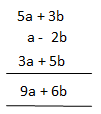(ii)(iii)(iv)(v)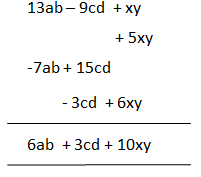(vi)3. Find the total savings of a boy who saves ₹ (4x – 6y); ₹ (6x + 2y); ₹(4y – x) and ₹ (y – 2x) for four consecutive weeks.
Solution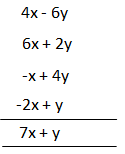∴ Total savings = ₹(7x + y)

4. Subtract :
(i) 4xy2 from 3xy2
(ii) -2x2y + 3xy2 from 8x2y :
(iii) 3a – 5b + c + 2d from 7a – 3b + c – 2d
(iv) x3 – 4x – 1 from 3x2 – x2 + 6
(v) 6a + 3 from a3 – 3a2 + 4a + 1
(vi) cab – 4cad – cbd from 3abc + 5bcd – cda
(vii) a2 + ab + b2 from 4a2 – 3ab + 2b2
Solution
(i) 3xy2 – 4xy2 = -xy2

(ii)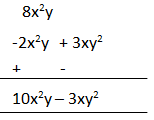(iii)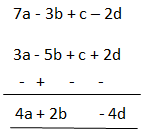(iv)(v)(vi)(vii)5. (i) Take away – 3x3 + 4x2 – 5x + 6 from 3xy3 – 4x2 + 5x – 6
(ii) Take m2 + m + 4 from - m2 + 3m + 6 and the result from m2 + m + 1.
Solution
(i)(ii)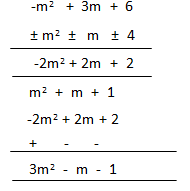6. Subtract the sum of 5y2 + y – 3 and y2 – 3y + 7 from 6y2 + y – 2.
Solution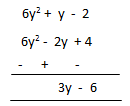7. What must be added to x4 – x3 + x2 + x + 3 to obtain x4 + x2 – 1?
Solution8. (i) How much more than 2x2 + 4xy + 2y2 is 5x2 + 10xy – y2 ?
(ii) How much less 2a2 + 1 is than 3a2 – 6 ?
Solution
(i)(ii)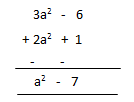9. If x = 6a + 86 + 9c ; y = 2b – 3a – 6c and z = c – b + 3a ; find
(i) x + y + z
(ii) x – y + z
(iii) 2x – y – 3z
(iv) 3y – 2z – 5x
Solution
(i)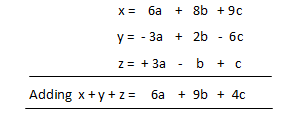(ii) x – y + z = (6a + 8b + 9c) – (2b – 3a – 6c) + (c – b + 3a)
= 6a + 8b + 9c – 2b + 3a + 6c + c – b + 3a
= 6a + 3a + 3a + 8b – 2b – b + 9c + 6c + c
= 12a + 5b + 16c

(iii) 2x – y – 3z
= 2(6a + 8b + 9c) – (2b – 3a – 6c) – 3(c – b + 3a)
= 12a + 16b + 18c – 2b + 3a + 6c – 3c + 3b – 9a
= 12a + 3a – 9a + 16b + 3b – 2b + 18c + 6c – 3c
= 6a + 17b + 21c

(iv) 3y – 2z – 5x
= 3(2b – 3a – 6c) – 2(c – b + 3a) – 5(6a + 8b + 9c)
= 6b – 9a – 18c – 2c + 2b – 6a – 30a – 40b – 45c
= - 9a – 6a – 30a + 6b + 2b – 40b – 18c – 2c – 45c
= - 45a – 32b – 65c

10. The sides of a triangle are x2 – 3xy + 8, 4x2 + 5xy – 3 and 6 – 3x2 + 4xy. Find its perimeter.
Solution
Required perimeter = Sum of three sides
= x2 – 3xy + 8 + 4x2 + 5xy – 3 + 6 – 3x2 + 4xy
= x2 + 4x2 – 3x2 – 3xy + 5xy + 4xy + 8 – 3 + 6
= 2x2 + 6xy + 11

11. The perimeter of a triangle is 8y2 – 9y + 4 and its two sides are 3y– 5y and 4y + 12. Find its third side.
Solution
Perimeter of the triangle = Sum of three sides
= 8y– 9y + 4
Sum of two sides = 3y – 5y + 4y + 12
= 7y – 5y + 12
∴ (8y – 9y + 4) – (7y – 5y + 12)
= 8y – 9y + 4 – 7y + 5y – 12
= y – 4y – 8
Hence third side = y – 4y – 8

12. The two adjacent sides of a rectangle are 2x – 5xy + 3z and 4xy – x – z. Find its perimeter.
Solution
Adjacent sides of a rectangle are
2x2 – 5xy + 3zand 4xy – x– z
∴ Perimeter = 2(2x– 5xy + 3z+ 4xy – x – z)
= 4x– 10xy + 6z+ 8xy – 2x – 2z
= 2x– 2xy + 4z

13. What must be subtracted from 19x4 + 2x+ 30x – 37 to get 8x+ 22x3 – 7x – 60 ?
Solution
The required result will be
(19x4 + 2x3 + 30x – 37) – (8x+ 22x3 – 7x – 60)
= 19x4 + 2x3 + 30x – 37 – 8x4 – 22x+ 7x + 60
= 11x4 – 20x3 + 37x + 23

14. How much smaller is 15x – 18y + 19z than 22x – 20y – 13z + 26 ?
Solution
The required result is
(22x – 20y – 13z + 26) – (15x – 18y + 19z)
= 22x – 20y – 13z + 26 – 15x + 18y – 19z
= 7x – 2y – 32z + 26

15. How much bigger is 15x2y2 – 18xy– 10x2y than - 5x+ 6x2y – 7xy ?
Solution
The required result,
(5x2y– 18xy- 10x2y) – (-5x+ 6x2y – 7xy)
= 5x2y– 18xy– 10x2y + 5x2 + 6x2y + 7xy
= 5x2y2 – 18xy– 16x2y + 5x+ 7xy

### Exercise 11 C

1. Multiply:
(i) 8ab2 by – 4a3b4
(ii) 2/3ab by -1/4a2b
(iii) -5cd2  by -5cd2
(iv) 4a and (6a + 7)
(v) -8x and (4 – 2x – x2)
(vi) 2a2 – 5a – 4 and -3a
(vii) x + 4 by x - 5
(viii) 5a – 1 by 7a – 3
(ix) 12a + 5b by 7a – b
(x) x2 + x + 1 by 1 – x
(xi) 2m2 – 3m – 1 and 4m2 – m – 1
(xii) a2, ab and b2
(xiii) abx, -3a2x and 7b2y2
(xiv) -3bx, -5xy and -7b3y2
(xv) (-3/2x5y3) and (4/9a2x3y)
(xvi) (-2/3a7b2) and (-9/4ab5)
(xvii) (2a– 3a2b) and (-1/2 ab2)
(xviii) (2x + 1/2y) and (2x – 1/2y)
Solution
(i) 8ab2 × -4a3b4
= (8 ×-4)(ab2 × a3b4)
= -32a1+3. b2+4
= -32a4b6

(ii) 2/3ab × -1/4a2b
= (2/3 × -1/4) (ab × a2b)
= -1/6a1+2. b1+1
= -1/6a3b2

(iii) -5cd2 × -5cd2
= (-5 × -5)(cd× cd2)
= 25c1+1. d2+2
= 25c2d4

(iv) 4a (6a + 7)
= 4a × 6a + 4a × 7
= 24a2 + 28a

(v) -8x (4 – 2x – x2)
= -8x×4 – 8x×-2x – 8x×x2
= -32x + 16x2 + 8x3

(vi) -3a(2a2– 5a – 4)
= -3a×2a2 – 5a×-3a – 4×-3a
= -6a3 + 15a2 + 12a

(vii) (x + 4)(x – 5)
= x(x – 5) + 4(x – 5)
= x2 – 5x + 4x – 20
= x2 – x – 20

(viii) (5a – 1)(7a – 3)
= 5a (7a – 3) – 1(7a – 3)
= 35a2 – 15a – 7a + 3
= 35a2 – 22a + 3

(ix) (12a + 5b)(7a – b)
= 12a(7a – b) + 5b (7a – b)
= 84a2 – 12ab + 35ab – 5b2
= 84a2 + 23ab – 5b2

(x) (x2 + x + 1)(1 – x)
= 1(x2 + x + 1) – x(x2 + x + 1)
= x2 + x + 1 – x3 – x2 – x
= 1 – x3

(xi) (2m2 – 3m – 1) (4m2 – m – 1)
= 2m2  (4m – m - 1) – 3m(4m2 – m – 1) – 1(4m2 – m – 1)
= 8m4 – 2m3 – 2m2 – 12m3 + 3m2 + 3m – 4m2 + m + 1
= 8m4 – 14m3 – 6m2 + 3m2 + 4m + 1
= 8m4 -  14m3 – 3m2  + 4m + 1

(xiii) a2 × ab × b2
= a2+1. b1+2
= a3b3

(xiii) abx × -3a2x × 7b2x
= (-3 × 7)(a × a2) (b × b2)(x × x × x3)
= -21a3b3x5

(xiv) -3bx × -5xy × -7b3y2
= (-3 × -5 × -7)(b × b3)(x × x)(y × y2)
= -105b4x2y3

(xv) (-3/2x5y3)(4/9a2x3y)
= (-3/2 × 4/9)(a2)(x× x3)(y3 × y)
= -2/3 a2x8y4

(xvi) (-2/3a7b2)(-9/4ab5)
= (-2/3 × -9/4)(a× a)(b2 × b5)
= 3/2a8b7

(xvii) (2a3 – 3a2b)(-1/2ab2)
= -1/2ab2(2a3 – 3a2b)
= 2a3 × -1/2ab2 – 3a2b × -1/2ab2
= -a4b2 + 3/2a3b3

(xviii) (2x + 1/2y)(2x – 1/2y)
= 2x(2x – 1/2y) + 1/2y(2x – 1/2y)
= 4x2 – xy + xy – 1/4y2
= 4x2 – 1/4y2

2. Multiply :
(i) 5x2 – 8xy + 6y2 – 3 by – 3xy
(ii) 3 – 2/3xy + 5/7xy2 – 16/21x2y by -21x2y2
(iii) 6x3 – 5x + 10 by 4 – 3x2
(iv) 2y - 4y3 + 6y5 by y2 + y – 3
(v) 5p2 + 25pq + 4q2 by 2p2 - 2pq + 3q2
Solution
(i) 5x2 – 8xy + 6y2 – 3×-3xy
= 15x3y3 + 24x2y2 – 18xy3 + 9xy

(ii)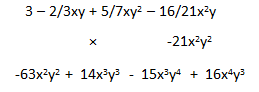(iii)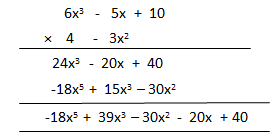(iv)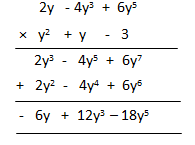(v)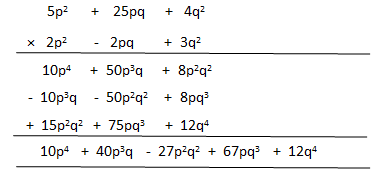3. Simplify :
(i) (7x – 8) (3x + 2)
(ii) (px – q) (px + q)
(iii) (5a + 5b – c) (2b – 3c)
(iv) (4x – 5y) (5x – 4y)
(v) (3y + 4z) (3y – 4z) + (2y + 7z) (y + z)
Solution
(i) (7x – 8)(3x + 2)
= 7x(3x + 2) – 8(3x + 2)
= 21x2 + 14x – 24x – 16
= 21x2 – 10x – 16

(ii) (px – q)(px + q)
= px(px + q) – q(px + q)
= p2x2 + pxq – pqx – q2
= p2x2 – q2

(iii) (5a + 5b – c)(2b – 3c)
= 5a(2b – 3c) + 5b(2b – 3c) – c(2b – 3c)
= 10ab – 15ac + 10b2 – 15bc – 2bc + 3c2
= 10ab + 10b2 – 17bc – 15ac  + 3c2

(iv) (4x – 5y)(5x – 4y)
= 4x(5x – 4y) – 5y(5x – 4y)
= 20x2 – 16xy – 25xy  + 20y2
= 20x2 – 41xy  + 20y2

(v) (3y + 4z)(3y – 4z) + (2y + 7z)(y + z)
= 3y(3y – 4z) + 4z(3y – 4z) + 2y(y + z) + 7z(y + z)
= 9y2 – 12yz + 12yz – 16z2 + 2y2 + 2yz + 7yz + 7z2
= (9 + 2)y2 + (-12 + 12 + 2 + 7)yz + (-16 + 7)z2
= 11y2 + 9yz – 9z2

4. The adjacent sides of a rectangle are x2 – 4xy + 7y2 and x3 – 5xy2. Find its area.
Solution
Reqd. area = (x2 – 4xy + 7y2)(x3 – 5xy2)
= x2(x3 – 5xy2) – 4xy(x3 – 5xy2) + 7y2(x3 + 5xy2)
= x5 – 5x3y2 – 4x4y + 20x2y3 + 7x3y2 – 35xy4
= x5 + (7 – 5)x3y2 – 4x4y + 20x2y3 – 35xy4
= x5 + 2x3y2 – 4x4y + 20x2y3 – 35xy4
= (x5 – 4x4y + 2x3y2 + 20x2y3 – 35xy4)sq. unit

5. The base and the altitude of a triangle are (3x – 4y) and (6x + 5y) respectively. Find its area.
Solution
Reqd. Area = 1/2 (base)× (altitude)
= ½ (3x – 4y)(6x + 5y)
= ½ (18x2 + 15xy – 24xy – 20y2)
= ½ (18x2 – 9xy  - 20y2) sq. unit

6. Multiply -4xy3 and 6x2y and verify your result for x = 2 and y= 1.
Solution
(-4xy3) × (6x2y)
= (-4 × 6)(x × x2)(y3 × y)
= -24x3y4
For x = 2 and y = 1
(-4xy3)× (6x2y)
= (-4×2×13) × (6×22×1)
= (-8) × 24
= -192
And, -24x3y4 = -24 × 23 × 14
= -24 × 8 × 1
= -192
∴ For x = 2 and y = 1, it is verified that
(-4xy3) × (6x2y)
= -24x3y4

7. Find the value of (3x3) x (-5xy2) x (2x2yz3) for x = 1, y = 2 and z = 3.
Solution
For x = 1, y = 2 and z = 3
(3x3) × (-5xy2) × (2x2yz3)
(3 × 13) × (-5 × 1 × 22) × (2 × 12 × 2 × 33)
= 3 × (-5 × 4) × (2 × 1 × 2 × 27)
= 3 × (-20) × 108
= -6480

8. Evaluate (3x4y2) (2x2y3) for x = 1 and y = 2.
Solution
(3x4y2)(2x2y3)
(3 × 14 × 22) × (2 × 12 × 23)
= (3 × 1 × 4) × (2 × 1 × 8)
= 12 × 16
= 192

9. Evaluate (x5) x (3x2) x (-2x) for x = 1.
Solution
For x = 1
(x5) × (3x2) × (-2x)
(15) × (3 × 12) × (-2 × 1)
= 1 × 3 × (-2)
= -6

10. If x = 2 and y = 1; find the value of (-4x2y3) x (-5x2y5).
Solution
For x = 2 and y = 1
(-4x2y3) × (-5x2y5)
= (-4 × 22 × 13) × (-5 × 22 × 15)
= (-4 × 4 × 1) × (-5 × 4 × 1)
= -16 × -20
= 320

11. Evaluate:
(i) (3x – 2)(x + 5) for x = 2.
(ii) (2x – 5y)(2x + 3y) for x = 2 and y = 3.
(iii) xz (x2 + y2) for x = 2, y = 1 and z= 1.
Solution
(i) For x = 2
(3x – 2)(x + 5)
= (3 × 2 – 2)(2 + 5)
= (6 – 2) × 7
= 4 × 7
= 28

(ii) For x = 2 and y = 1
xy2(x – 5y) +1
2 × 12(2 – 5 × 1) + 1
= 2 × (2 – 5) + 1
= 2 × (-3) + 1
= -6 + 1
= -5

(iii)
For x = 2, y = 1 and z = 1
xz(x2 + y2)
= 2 × 1(22 + 12)
= 2(2 + 1)
= 2 × 3
= 6

12. Evaluate:
(i) x(x – 5) + 2 for x = 1.
(ii) xy2(x – 5y) + 1 for x = 2 and y = 1.
(iii) 2x(3x – 5) – 5(x – 2) – 18 for x = 2.
Solution
(i) For x =1
x(x – 5) + 2
= 1(1 – 5) + 2
= -4 + 2
= -2

(ii) For x = 2 and y = 1
xy2(x – 5y)
= 2 × 12(2 – 5 × 1)
= 2 × (2 – 5)
= 2 × (-3)
= -6

(iii) For x = 2
2x(3x – 5) – 5(x – 2) – 18
= 2 × 2(3 × 2 -  5) – 5(2 – 2) – 18
= 4(6 – 5) – 5 × 0 – 18
= 4 – 18
= -14

13. Multiply and then verify :
-3x2y2 and (x – 2y) for x = 1 and y = 2.
Solution
(-3x2y2) × (x – 2y)
= (-3x2y2) × (x) – (-3x2y2)(2y)
= - 3x3y2 + 6x2y3
= 6x2y3 – 3x3y2
For x = 1 and y = 2
(-3x2y2) × (x – 2y)
= (-3 × 12 × 22) × (1 - 2 × 2)
= (6 × 1 × 8) - (3 × 1 × 4)
= 48 - 12
= 36
∴ For x = 1 and y = 2, it is verified that,
(-3x2y2) × (x – 2y)
= 6x2y3 – 3x3y2

14. Multiply:
(i) 2x2 – 4x + 5 by x2 + 3x – 7
(ii) (ab – 1)(3 – 2ab)
Solution
(i) 2x2 – 4x + 5 by x2 + 3x – 7
= (2x– 4x + 5) × (x2 + 3x – 7)
= 2x2(x2 + 3x - 7) – 4x(x2 + 3x – 7) + 5(x2 + 3x – 7)
= 2x4 + 6x3 – 14x2 – 4x3 – 12x2 + 28x + 5x2 + 15x – 35
= 2x4 + 6x3 – 4x3 – 14x2 – 12x2 + 5x2 + 28x + 15x – 35
= 2x4 + 2x3 – 21x2 + 43x – 35

(ii) (ab - 1)(3 – 2ab)
= ab(3 – 2ab) – 1(3 – 2ab)
= 3ab - 2a2b2 – 3 + 2ab
= -2a2b2 + 5ab – 3
= 2a2b2 – 5ab + 3

15. Simplify : (5 – x)(6 – 5x)(2 - x).
Solution
(5 – x)(6 – 5x)(2 – x)
= [(5 – x)(6 – 5x)](2 – x)
= [5(6 – 5x) – x(6 – 5x)] (2 – x)
= [30 – 25x – 6x + 5x2](2 – x)
= (5x2 – 31x + 30)(2 – x)
= 2(5x2 – 31x + 30) - x(5x2 – 31x + 30)
= 10x2 - 62x + 60 – 5x3 + 31x2 – 30x
= -5x3 + 10x2 + 31x2 – 62x – 30x + 60
= -5x3 + 41x2 – 92x + 60

### Exercise 11 D

1. Divide :
(i) -70a3 by 14a2
(ii) 24x3y3 by -8y2
(iii) 15a4b by -5a3b
(iv) -24x4d3 by -2x2d5
(v) 63a4b5c6 by -9a2b4c3
(vi) 8x – 10y + 6c by 2.
(vii) 15a3b4 – 10a4b3 – 25a3b6 by -5a3b2
(viii) -14x6y3 – 21x4y5 + 7x5y4 by 7x2y2
(xi) 12x2 + 7xy – 12y2 by 3x + 4y
(xii) x6 – 8 by x2 – 2
(xiii) 6x3 – 13x2 – 13x + 30  by 2x2 - x - 6
(xiv) 4a2 + 12ab + 9b2 - 25c2 by 2a + 3b + 5c .
(xv) 16 + 8x + x6 - 8x3- 2x4 + x2 by x + 4 - x3
Solution
(i) -70a3/14a2
= (-70/14)(a3/a2)
= -5a3-2
= -5a

(ii) 24x3y3/-8y2
= (24/-8)(x3)(y3/y2)
= -  3x3y3-2
= -3x3y

(iii) 15a4b/-5a3b
= (15/-5)(a4/a3)(b/b)
= -3a4-3b1-1
= -3a b0
= -3a × 1 (∵ b0 = 1)
= -3a

(iv) (- 24x4d3/-2x2d5)
= (-24/-2)(x4/x2)(d3/d5)
= 12x4-2dx3-5
= 12x2d-2
= 12x2/d2

(v) 63a4b5c6/-9a2b4c3
= (63/-9)(a4/a2)(b5/b4)(c6/c3)
= -7a4-2. b5-4. c6-3
= -7a4-2. b5-4. c6-3
= -7a2bc3

(vi) (8x – 10y + 6c)/2
= 8x/2 – 10y/2 + 6c/2
= 4x – 5y + 3c

(vii) (15a3b4 – 10a4b3 – 25a3b6)/-5a3b2
= 15a3b4/-5a3b2 – 10a4b3/-5a3b2 – 25a3b6/-5a3b2
= -3b4-2 + 2a4-3b3-2 + 5b6-2
= -3b2 + 2ab + 5b4

(viii) (-14x6y3 – 21x4y5 + 7x5y4)/7x2y2
= -14x6y3/7x2y2 – 21x4y5/7x2y2 + 7x5y4/7x2y2
= -2x6-2y3-2. -3x4-2y5-2 + x5-2y4-2
= -2x4y – 3x2y3 + x3y2

(ix)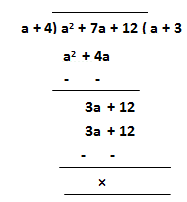∴ Answer = a + 3

(x)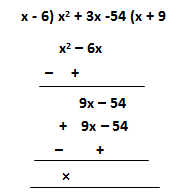∴ Answer = x + 9

(xi)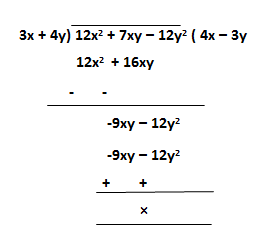∴ Answer = 4x - 3y

(xii)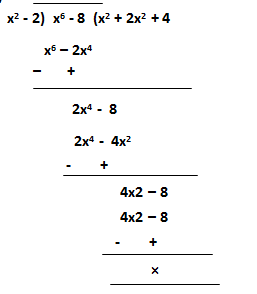∴ Answer = x4 + 2x2 + 4

(xiii)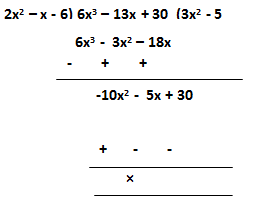∴ Answer = 3x – 5

(xiv)∴ Answer = 2a + 3b – 5c

(xv)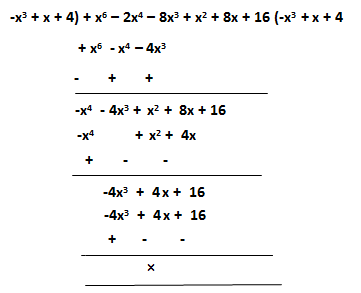∴ Answer = -x3 + x + 4 + 3b

2. Find the quotient and the remainder (if any) when :
(i) a3 – 5a2 + 8a + 15 is divided by a + 1.
(ii) 3x4 + 6x3 – 6x2 + 2x – 7 is divided by x – 3.
(iii) 6x2 + x – 15 is divided by 3x + 5.
(iv) 6y5 + 30y4 + 18y3 + 6y2 + 15y + 3 is divided by 2y3 + 1.
Solution
(i)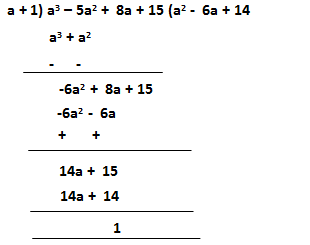∴ Quotient = a2 – 6a + 14 and remainder = 1
Verification:
Dividend = Quotient × Divisor + Remainder
= (a2 – 6a + 14) × (a + 1) + 1
= a3 – 6a2 + 14a + a2 – 6a + 14 + 1
= a2 – 5a2 + 8a + 15 which is given

(ii)∴ Quotient = 3x2 + 15x2 + 39x + 119 and remainder = 350
Verification:
Dividend = Quotient × Divisor × Remainder
= (3x3 + 15x2 + 39x + 119)(x – 3) + 350
= 3x4 + 15x3 + 39x2 + 119x – 9x3 – 45x2 – 117x – 357 + 350
= 3x4 + 6x3 – 6x2 + 2x – 7 which is given

(iii)∴ Quotient = 2x – 3 and remainder = 0
Verification:
Dividend = Quotient × Divisor  + Remainder
= (2x – 3)(3x + 5) + 0
= 6x2 + 10x – 9x – 15 + 0
= 6x2 – x – 15 which is given

(iv)∴ Quotient = 3y2 + 15y + 9 = 0 and remainder = 3y2 – 6
Verification:
Dividend = Quotient × Divisor + Remainder
= (3y2 + 15y + 9)(2y3 + 1) + 3y2 – 6
= 6y5 + 30y4 + 18y3 + 3y2 + 15y + 9 + 3y2 – 6
= 6y5 + 30y4 + 18y3 + 6y2 + 15y + 3 which is given

3. The area of a rectangle is x3 – 8x2 + 7 and one of its sides is x – 1. Find the length of the adjacent side.
Solution
Area = x+ 7
One side = x – 1
∴ Adjacent side = (x3 – 8x2 + 7) + (x – 1)∴ Other side = x2 – 7x – 7

4. The product of two numbers is 16x4 – 1. If one number is 2x – 1, find the other.
Solution
Product of two numbers = 16x4 – 1
One number = 2x – 1
Then second number = (16x4 – 1)/(2x – 1)
= 8x3 + 4x2 + 2x + 15. Divide x6 – y6 by the product of x2 + xy + y2 and x – y.
Solution
Product of (x2 + xy + y2) and (x – y)
= (x – y)(x2 + xy + y2)
= x(x2 + xy + y2) – y(x2 + xy + y2
= x3 + x2y + xy2 – x2y – xy2 – y3
= x3 – y3
Now, (x6 – y6) ÷ (x3 – y3)
= x3 + y3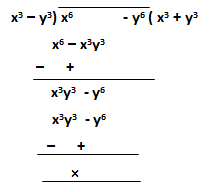### Exercise 11 E

1. a2 – 2a + {5a2 – (3a – 4a2)}

Solution

a2 – 2a + {5a2 – 3a + 4a2}
= a2 – 2a + {9a – 3a}
= a2 – 2a + 9a2 – 3a
= 10a2 – 5a

2.Solution= x – y – {x – y – (x + y) – x + y}
= x – y – {x – y – x – y – x + y}
= x – y – x + y + x + y + x – y
= 2x

3. -3(1 – x) {x2 – (3 – 2x2)}
Solution
-3(1 – x2) – 2{x2 – (3 – 2x2)}
= -3 + 3x2 – 2 {x2 – 3 + 2x2}
= -3 + 3x2 – 2{3x2 – 3}
= -3 + 3x2 – 2{3x2 – 3}
= -3 + 3x2 – 6x2 + 6
= 3 – 3x2

4.Solution= 2{m – 3(n + m – 2n)}
= 2{m – 3 (m – n)}
= 2{m – 3m + 3n}
= 2{3n – 2m}
= 6n – 4m

5.Solution= 3x – [3x – {3x – (3x – 3x + y)}]
= 3x – [3x – {3x – y}]
= 3x – [3x – 3x + y]
= 3x – y

6.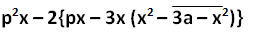Solution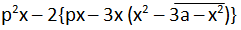= p2x – 2{px – 3x(x2 – 3a + x2)}
= p2x – 2{px – 3x(2x2 – 3a)}]
= p2x – 2{px + 6x3 + 9ax}
= p2x – 2px + 12x3 – 18ax

7.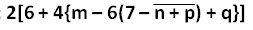Solution= 2[6 + 4{m – 6 (7 – n – p) + q}]
= 2[6 + 4{m – 42 + 6n + 6p + q}]
= 2[6 + 4m - 168 + 24n + 24p +  4q]
= 2[4m + 24n + 24p + 4q – 162]
= 8m + 48n + 48p + 8q – 324

8.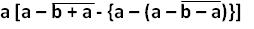Solution= a -  [a – b – a – {a – (a – b + a)}]
= a – [- b – {a – a + b – a}]
= a – [- b  – b + a]
= a + b + b – a
= 2b

9.Solution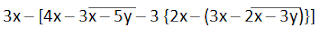= 3x – [4x – 3x + 5y – 3{2x – (3x – 2x + 3y)}]
= 3x – [4x – 3x + 5y – 3 {2x – (x + 3y)}]
= 3x – [4x – 3x + 5y – 3{2x – x – 3y}]
= 3x – [x + 5y – 6x + 3x + 9y]
= 3x – [-2x + 14y]
= 3x + 2x – 14y
= 5x – 14y

10. a5 ÷ a3 ÷ 3a × 2a
Solution
a5 ÷ a3 + 3a × 2a
= a5-3 + 3a × 2a
= a2 + 6a2
= 7a2

11. x5 ÷ (x2 × y2) × y3
Solution
x5 ÷ (x2 × y2) × y3
= x5/x2y2 × y3
= x5-2 – y3-2
= x3y

12. (x5 ÷ x2) × y2 × y3
Solution
(x5 ÷ x2) × y2 × y3
= x5-2 × y2+3
= x3y5

13. (y3 – 5y2) ÷ y × (y - 1)
Solution
(y3 – 5y2) ÷ y × (y – 1)
= (y3 – 5y2)/y × (y – 1)
= (y2 – 5y) × (y – 1)
= y2(y – 1) – 5y(y – 1)
= y3 – y2 – 5y2 + 5y
= y3 – 6y2 + 5y

14.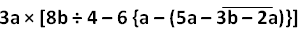Solution= 3a × [8b/4 – 6{a – (5a – 3b + 2a)}]
= 3a × [2b – 6 {a – 7a + 3b}]
= 3a × [2b – 6 {-6a + 3b}]
=  3a × [2b + 36a – 18b]
= 3a × [36a – 16b]
= 108a2 – 48ab

15. 7x + 4{x2 ÷ (5x ÷ 10)} – 3{2 – x3 ÷ (3x2 ÷ x)}
Solution
7x + 4{x2 ÷ (5x ÷ 10)} – 3{2 – x3 ÷ (3x2 ÷ x)}
= 7x + 4{x2 ÷ (5x/10)} – 3{2 – x3 ÷ (3x2/x)}
= 7x + 4 {x2 ÷ x/2} – 3{2 – x3 ÷ 3x}
= 7x + 4{x2 × 2/x} – 3{2 – x3/3x}
= 7x + 8x – 6 + x2
= x2 + 15x – 6